# Logical Reasoning for Job Interviews - Set 2

1)   In the following question, choose the correct code form.

If ACID is written as 1C3D, PAMPER is written as P1MP2R, then how will BOMBAY be written?

a. B4MB1Y
b. B3MB1Y
c. B5MB2Y
d. B4MB2Y
 Answer  Explanation ANSWER: B4MB1Y Explanation: Vowels A, E, I, O, U are coded as 1, 2, 3, 4, 5 respectively.Each of the consonants is kept same.So, the code for BOMBAY becomes B4MB1Y

2)   In the following question, choose the correct code form.

If ANY = 40, MANY = 53 then MANIAC = ?

a. 41
b. 42
c. 43
d. 44
e. 45
 Answer  Explanation ANSWER: 41 Explanation: In the given code, A = 1, B = 2, C = 3 and so on till Z =26.So, MANIAC = 13 + 1 + 14 + 9 + 1 + 3 = 41

3)   16 teams participated in a cricket tournament.

The tournament was conducted in two stages.

First stage
• In the first stage, the teams are divided into two equal groups.
• Each team plays every other team in its group exactly once.
• At the end of first stage, the top four teams from each group advance to the second stage.
• Rest teams are eliminated.
Second stage
• The second stage comprised several rounds.
• A round involves one match for each team. The winner of a match in a round advances to the next round, while the loser is eliminated.
• The team that remains undefeated in the second stage is declared the winner and claims the Gold Cup.
Rules
• The tournament rules such that each match results in a winner and a loser with no possibility of a tie.
• In the first stage, a team earns one point for each win and no points for a loss.
• At the end of the first stage, teams in each group are ranked on the basis of total points to determine the qualifiers advancing to the next stage.
• Ties are resolved by a series of complex tie-breaking rules so that exactly four teams from each group advanced to the next stage.
What would be the total number of matches in the tournament?

a. 55
b. 56
c. 63
d. 66
 Answer  Explanation ANSWER: 63 Explanation: In first stage, teams are divided into two groups of 8 teams each.They play a match against everyone exactly one i.e. 8C2 matches in every group. So, 2 x 8C2 = 56 matches for the first stage.8C2 = 8! / (2!)(6!) = 4 x 7 = 28In second stage, there are 8 teams in a “knockout stage”. There will be one winner, so 8 – 1 = 7So, total number of matches is 56 + 7 = 63

4)   Choose the odd pair of words

a. Profit - Loss
b. Virtue - Vice
c. Seduce - Attract
d. Wise - Foolish
 Answer  Explanation ANSWER: Seduce - Attract Explanation: In all other pairs, the words are antonyms of each other.

5)   Choose the odd pair.

a. Quran – Islam
b. Tripitika - Buddhism
c. Kojiki - Shintoism
d. Temple - Hindu
 Answer  Explanation ANSWER: Temple - Hindu Explanation: In all of the other pairs, first is the sacred and religious book of the second.

• Six cute dogs Abby, B-dawg, Charlie, Dalton, Eddie and Fantom are standing in two rows, three in each.
• Eddie is not at the end of any row.
• Dalton is second to the left of Fantom.
• Charlie, neighbor of Eddie, is standing diagonally opposite to Dalton.
• B-dawg is the neighbor of Fantom.
Which of the following are standing diagonally opposite to each other?

a. Fantom and Charlie
b. Dalton and Abby
c. Abby and Fantom
d. Abby and B-dawg

Explanation:
Eddie is not at the end, so, Eddie must be in the middle of one of the rows.

Dalton is second to the left of Fantom i.e. Dalton, ___, Fantom

Charlie is the neighbor of Eddie and is standing diagonally opposite to Dalton.

B-dawg is the neighbor of Fantom.

So, the arrangement is –

 Dalton B-dawg Fantom Abby Eddie Charlie
Other than Dalton and Charlie (given in statements), Abby and Fantom are diagonally opposite to each other.

• Six cute dogs Abby, B-dawg, Charlie, Dalton, Eddie and Fantom are standing in two rows, three in each.
• Eddie is not at the end of any row.
• Dalton is second to the left of Fantom.
• Charlie, neighbor of Eddie, is standing diagonally opposite to Dalton.
• B-dawg is the neighbor of Fantom.
Which of the following are not in the same row?

a. Abby and Eddie
b. Eddie and Charlie
c. Dalton and B-dawg
d. Abby and B-dawg

Explanation:
Eddie is not at the end, so, Eddie must be in the middle of one of the rows.

Dalton is second to the left of Fantom i.e. Dalton, ___, Fantom

Charlie is the neighbor of Eddie and is standing diagonally opposite to Dalton.

B-dawg is the neighbor of Fantom.

So, the arrangement is –

 Dalton B-dawg Fantom Abby Eddie Charlie

We can see clearly from the final arrangement that Abby and B-dawg are not in the same row.

8)   In the following question, one term in the number series is wrong. Find the wrong term.

125, 126, 124, 127, 122, 128

a. 122
b. 124
c. 125
d. 126
 Answer  Explanation ANSWER: 122 Explanation: The correct sequence is – +1 -2 +3 -4 +5 -6 The wrong number is 122The correct number should be 127 - 4 = 123

9)   In the following question, one term in the number series is wrong. Find the wrong term.

1, 3, 10, 21, 63, 129

a. 10
b. 21
c. 63
d. 129
 Answer  Explanation ANSWER: 63 Explanation: The pattern in the give series is – x 2 + 1, x 3 + 1, x 2 + 1, x 3 + 1The wrong number is 63The correct number should be: (21 x 3) + 1 = 64

10)   In the question below is given a statement followed by two courses of action numbered I and II. You have to assume everything in the statement to be true and based on the information given in the statement, decide which of the suggested courses of action logically follow(s) for pursuing.

(A) If only I follows
(B) If only II follows
(C) If either I or II follows
(D) If neither I nor II follow
(E) If both I and II follow.

Statement - The trader’s union feel that the prices of tomatoes will go up and lead to black-marketing.

Courses of Action - I. The Government should exercise a stringent law against hoarders and black-marketers.

II. Large network of fair price shops for the sale of tomatoes should be established by the Government.

a. Only I follows
b. Only II follows
c. Either I or II follows
d. Neither I nor II follow
e. Both I and II follow
 Answer  Explanation ANSWER: Both I and II follow Explanation: Both the courses of action can be taken to prevent shortage of tomatoes and black-marketing during the period of shortage. Here, both the courses should be followed.

11)   In the question below is given a statement followed by two courses of action numbered I and II. You have to assume everything in the statement to be true and based on the information given in the statement, decide which of the suggested courses of action logically follow(s) for pursuing.

(A) If only I follows
(B) If only II follows
(C) If either I or II follows
(D) If neither I nor II follow
(E) If both I and II follow.

Statement - The Japanese petroleum industry venturing into the west European markets faces tough competition from the Chinese.

Courses of Action - I. Japan should search for other markets for its products.

II. Japan should improve the quality of the petroleum products to compete with the Chinese in capturing these markets.

a. Only I follows
b. Only II follows
c. Either I or II follows
d. Neither I nor II follow
e. Neither I nor II follow
 Answer  Explanation ANSWER: Only II follows Explanation: Running away from the competition is not the solution in any case.Hence, Japanese should compete with the Chinese and emerge successful. So, only II follows.

12)   In the question below is given a statement followed by two conclusions numbered I and II.

(A) If only I follows
(B) If only II follows
(C) If either I or II follows
(D) If neither I nor II follows
(E) If both I and II follow.

Statement - If both the partners work as a team, marriage will be successful.

The couple celebrated golden jubilee of their wedding last year.

Conclusions - I. Both the partners are compatible with each other.

II. Some marriages do not last even ten years nowadays.

a. Only I follows
b. Only II follows
c. Either I or II follows
d. Neither I nor II follows
e. Both I and II follow
 Answer  Explanation ANSWER: Only I follows Explanation: The first statement asserts that a marriage will be successful if both partners work together. The second statement states that they celebrated their golden jubilee the previous year.Hence, only I follows while II does not.

13)   In the question below is given a statement followed by two conclusions numbered I and II.

(A) If only I follows
(B) If only II follows
(C) If either I or II follows
(D) If neither I nor II follows
(E) If both I and II follow.

Statement - High IQ is important to be a scientist.

Conclusions - I. The average student should not aspire to be a scientist.

II. All highly gifted students become scientist.

a. Only I follows
b. Only II follows
c. Either I or II follows
d. Neither I nor II follows
e. Both I and II follow
 Answer  Explanation ANSWER: Only I follows Explanation: Not all the intelligent students desire to be scientist. So, II does not follow. Hence, I follows.

• Two cars start from the opposite places of a main road, 300 km apart.
• First car runs for 50 km and takes a right turn and then runs 30 km.
• It then turns left and then runs for another 50 km and then takes the direction back to reach the main road.
• In the meantime, due to minor break down the other car has run only 70 km along the main road.
What would be the distance between two cars at this point?

a. 65 km
b. 70 km
c. 75 km
d. 130 km
 Answer  Explanation ANSWER: 130 km Explanation: Required Distance = DF = 300 – (50 + 50 + 70) = 130 km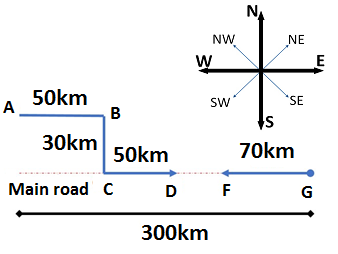15)   Karan is 100 m North-West of Lalit. If Manoj is 100 m North-East of Lalit, then Manoj is in which direction of Karan?

a. East
b. West
c. North
d. South
 Answer  Explanation ANSWER: East Explanation: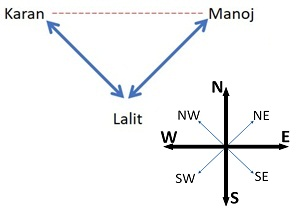There are six children playing football, namely, Z, Y, X, W, V and U.

1. Z and V are brothers.
2. U is the sister of V.
3. X is the only son of Z’s uncle.
4. Y and W are daughters of the brother of X’s father.

How many male players are there?

a. 3
b. 4
c. 5
d. 6
 Answer  Explanation ANSWER: 3 Explanation: As given, Z and V are brothers. Hence, both are males.U is the sister of V and hence female.X is the son and hence male.Y and W are daughters, hence female.Thus, there are three males.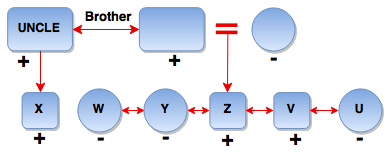There are six children playing football, namely, Z, Y, X, W, V and U.

1. Z and V are brothers.
2. U is the sister of V.
3. X is the only son of Z’s uncle.
4. Y and W are daughters of the brother of X’s father.

Which pair among the following is a pair of siblings?

a. X, Z
b. Z, V
c. X, W
d. U, X
 Answer  Explanation ANSWER: Z, V Explanation: As given, Z and V are brothers. Hence, they are siblings.While all other pairs are cousins.18)   In the question below is given a statement followed by two conclusions numbered I and II.

(A) If only I follows
(B) If only II follows
(C) If either I or II follows
(D) If neither I nor II follows
(E) If both I and II follow.

Statement - I. All dogs are cats.
II. All rats are cats.

Conclusion - I. No dogs are cat.
II. Some cat are rat.

a. Only I follows
b. Only II follows
c. Either I or II follows
d. Neither I nor II follows
e. Both I and II follow
 Answer  Explanation ANSWER: Only II follows Explanation: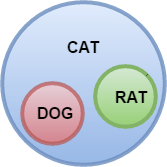• Superman began towards the Sun at 9 AM in the morning.
• After flying some distance, he turned to right and again to the right.
• After flying some distance, he again turned to the right.
In which direction is he flying now?

a. East
b. West
c. North
d. South
 Answer  Explanation ANSWER: North Explanation: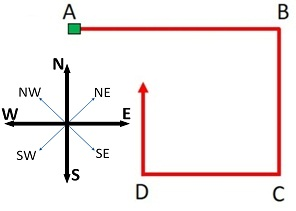20)   At what time between 9.13 and 10.12 will the hands of a watch be together?

a. 45 min past 9
b. 49(1/11) min past 9
c. 48 min past 9
d. 47 min past 9
 Answer  Explanation ANSWER: 49(1/11) min past 9 Explanation: To be together between 9 and 10 o'clock, the minute hand must gain 45 min. space55 min. spaces are gained in 60 min.45 min spaces would be gained in (60/55 × 45) min. or 49 1/11 min. The hands are together at 49 1/11 min. past 9.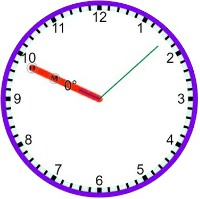There are six children playing football, namely, Z, Y, X, W, V and U.

1. Z and V are brothers.
2. U is the sister of V.
3. X is the only son of Z’s uncle.
4. Y and W are daughters of the brother of X’s father.

How is W related to Z?

a. Uncle
b. Cousin
c. Sister
d. Niece
 Answer  Explanation ANSWER: Cousin Explanation: W’s father is the brother of X’s father and X’s father is Z’s uncle.So, W’s father is also Z’s uncle.Thus, W is Z’s cousin.